## Example Questions

### Example Question #4 : Exponential Operations

Simplify:  y3x4(yx3 + y2x2 + y15 + x22)

y3x12 + y6x8 + y45x4 + y3x88

y4x7 + y5x6 + y18x4 + y3x26

y3x12 + y6x8 + y45 + x88

y3x12 + y12x8 + y24x4 + y3x23

2x4y4 + 7y15 + 7x22

y4x7 + y5x6 + y18x4 + y3x26

Explanation:

When you multiply exponents, you add the common bases:

y4 x7 + y5x6 + y18x4 + y3x26

### Example Question #5 : Exponential Operations

Indicate whether Quantity A or Quantity B is greater, or if they are equal, or if there is not enough information given to determine the relationship.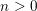Quantity A: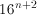Quantity B: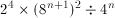Quantity B is greater.

The relationship cannot be determined from the information given.

Quantity A is greater.

The quantities are equal.

Quantity B is greater.

Explanation:

By using exponent rules, we can simplify Quantity B.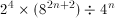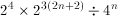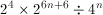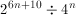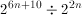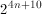Also, we can simplify Quantity A.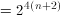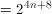Since n is positive,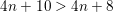### Example Question #6 : Exponential Operations

If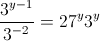, what is the value of?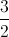Explanation:

Rewrite the term on the left as a product. Remember that negative exponents shift their position in a fraction (denominator to numerator).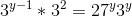The term on the right can be rewritten, as 27 is equal to 3 to the third power.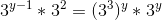Exponent rules dictate that multiplying terms allows us to add their exponents, while one term raised to another allows us to multiply exponents.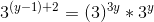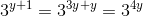We now know that the exponents must be equal, and can solve for.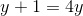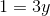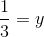### Example Question #11 : Exponential Operations

If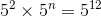, what is the value of?Explanation:

Since the base is 5 for each term, we can say 2 + n =12.  Solve the equation for n by subtracting 2 from both sides to get n = 10.

### Example Question #12 : Exponential Operations

Simplify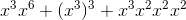.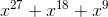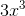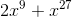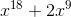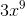Explanation:

Start by simplifying each individual term between the plus signs. We can add the exponents in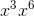and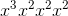so each of those terms becomes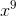. Then multiply the exponents in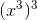so that term also becomes. Thus, we have simplified the expression to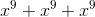which is.

### Example Question #451 : Algebra

Simplify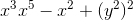.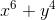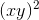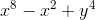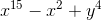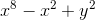Explanation:

First, simplify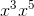by adding the exponents to get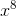.

Then simplify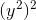by multiplying the exponents to get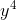.

This gives us. We cannot simplify any further.

### Example Question #14 : Exponential Operations

If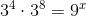, what is the value of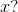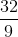Explanation:

To attempt this problem, note that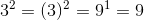.

Now note that when multiplying numbers, if the base is the same, we may add the exponents: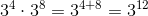This can in turn be written in terms of nine as follows (recall above)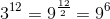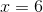### Example Question #15 : Exponential Operations

If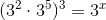, what is the value ofExplanation:

When dealing with exponenents, when multiplying two like bases together, add their exponents: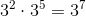However, when an exponent appears outside of a parenthesis, or if the entire number itself is being raised by a power, multiply: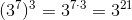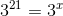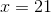Tired of practice problems?

Try live online GRE prep today.